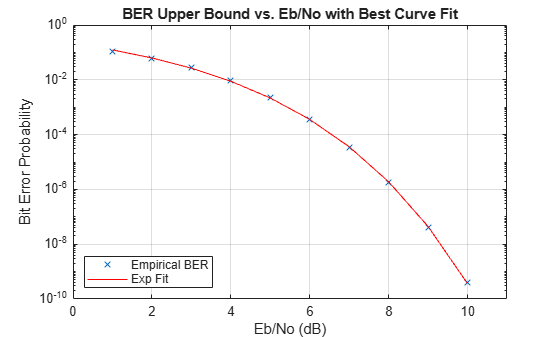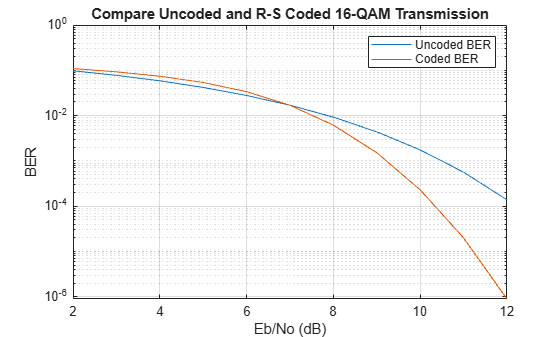# bercoding

BER for coded AWGN channels

## Syntax

``ber = bercoding(EbNo,'conv',decision,coderate,dspec)``
``ber = bercoding(EbNo,'block',decision,N,K,dmin)``
``ber = bercoding(EbNo,'Golay','hard',24)``
``ber = bercoding(EbNo,'Hamming','hard',N)``
``ber = bercoding(EbNo,'RS','hard',N,K) ``
``ber = bercoding(EbNo,coding,___,modulation)``

## Description

The `bercoding` function returns an upper bound or approximation of the bit error rate (BER) for coherent BPSK or QPSK modulation over an additive white Gaussian noise (AWGN) channel for a specified coding type, decoding decision, code rate, and distance spectrum of the code. The results for binary PSK and quadrature PSK modulation are the same. This function computes only modulation order 2 or 4 for M-ary PSK modulation. For more information, see Analytical Expressions Used in bercoding Function and Bit Error Rate Analysis App.

````ber = bercoding(EbNo,'conv',decision,coderate,dspec)` returns an upper bound or approximation of the BER for the convolutionally coded signal with the specified decoding decision, code rate, and distance spectrum of the code.```

example

````ber = bercoding(EbNo,'block',decision,N,K,dmin)` returns the upper bound of the BER for an [`N`,`K`] binary block code for the specified decoding decision type and minimum distance of the code.```
````ber = bercoding(EbNo,'Golay','hard',24)` returns the upper bound of the BER for an the extended (24, 12) Golay code using hard-decision decoding and coherent BPSK modulation. In accordance with , the Golay coding upper bound assumes only the correction of 3-error patterns. Even though correcting approximately 19% of 4-error patterns is theoretically possible in practice, most decoders do not have this capability.```
````ber = bercoding(EbNo,'Hamming','hard',N)` returns an approximation of the BER for a Hamming code using hard-decision decoding and coherent BPSK modulation.```
````ber = bercoding(EbNo,'RS','hard',N,K) ` returns an approximation of the BER for an (`N`,`K`) Reed-Solomon code using hard-decision decoding and coherent BPSK modulation.```

example

````ber = bercoding(EbNo,coding,___,modulation)` specifies a modulation type in addition to any of the previous input argument combinations. This syntax returns an approximation of the BER for coded AWGN channels.```

## Examples

collapse all

Find the upper bound of the theoretical BER for a (23,12) block code.

Set the codeword length, message length, minimum distance, and ${\mathit{E}}_{\mathrm{b}}/{\mathit{N}}_{0}$ range in dB.

```n = 23; % Codeword length k = 12; % Message length dmin = 7; % Minimum distance EbNo = 1:10; % Eb/No range (dB)```

Estimate the BER.

`berBlk = bercoding(EbNo,'block','hard',n,k,dmin);`

Plot the estimated BER.

```berfit(EbNo,berBlk) ylabel('Bit Error Probability') title('BER Upper Bound vs. Eb/No with Best Curve Fit')```Estimate the BER performance in an AWGN channel for a 16-QAM signal when encoded with a (15,11) Reed-Solomon code using hard-decision decoding.

Set the input Eb/No range and find the uncoded BER for 16-QAM.

```ebno = (2:12)'; uncodedBER = berawgn(ebno,'qam',16);```

Estimate the coded BER for a 16-QAM signal with a (15,11) Reed-Solomon code using hard-decision decoding.

`codedBER = bercoding(ebno,'RS','hard',15,11,'qam',16);`

Plot the estimated BER curves.

```semilogy(ebno,[uncodedBER codedBER]) grid title('Compare Uncoded and R-S Coded 16-QAM Transmission') legend('Uncoded BER','Coded BER') xlabel('Eb/No (dB)') ylabel('BER')```## Input Arguments

collapse all

Energy per bit to noise power spectral density ratio in dB, specified as a scalar or vector.

Data Types: `single` | `double`

Source coding type, specified as one of these options.

• `'conv'` — The returned BER is an upper bound of the BER for binary convolutional codes with coherent BPSK or QPSK modulation.

• `'block'` — The returned BER is an upper bound of the BER for (`N`, `K`) linear binary block codes with coherent BPSK or QPSK modulation.

• `'Hamming'` — The returned BER is an approximation of the BER for a Hamming code using hard-decision decoding and coherent BPSK or QPSK modulation.

• `'Golay'` — The returned BER is an upper bound of the BER for an extended (24,12) Golay code using hard-decision decoding and coherent BPSK or QPSK modulation. In accordance with , the Golay coding upper bound assumes the correction of 3-error patterns only. Even though correcting approximately 19% of 4-error patterns is theoretically possible, in practice, most decoders do not have this capability.

• `'RS'` — The returned BER is an approximation of the BER for an (`N`,`K`) Reed-Solomon code using hard-decision decoding and coherent BPSK modulation.

Data Types: `char` | `string`

Decoding decision type, specified as one of these options.

• `'hard'` — Use this option for hard-decision decoding.

• `'soft'` — Use this option for unquantized soft-decision decoding. This option applies only when `coding` is set to `'conv'` or `'block'`.

Data Types: `char` | `string`

Code rate of the convolutional code, specified as a positive scalar.

#### Dependencies

To enable this argument, set the `coding` argument to `'conv'`.

Data Types: `double` | `single`

Distance spectrum of the code, specified as structure containing these fields. To find distance spectra for sample codes, use the `distspec` function or see  and .

Minimum free distance of the code, specified as a positive scalar.

Data Types: `double` | `single`

Weight spectrum of the code, specified as a positive scalar.

Data Types: `double` | `single`

#### Dependencies

To enable this argument, set the `coding` argument to `'conv'`.

Data Types: `struct`

Codeword length, specified as an integer of the form 2M–1, where M is an integer in the range [3, 16]. For more information, see N-K Pairs for Source Coding.

Example: `15` or `2^4-1`

#### Dependencies

To enable this argument, set the `coding` argument to `'block'`, `'Hamming'`, `'Golay'`, or `'RS'`.

Message length, specified as a positive integer. For more information, see N-K Pairs for Source Coding.

Example: `5` specifies a Galois field array with five elements.

#### Dependencies

To enable this argument, set the `coding` argument to `'block'` or `'RS'`.

Minimum distance of the code, specified as a positive scalar smaller than or equal to (`N` - `K` + 1).

.

#### Dependencies

To enable this argument, set the `coding` argument to `'block'`.

Data Types: `double` | `single`

Modulation decision type, specified as `'psk'`, `'oqpsk'`, `'dpsk'`, `'pam'`, `'qam'`, `'fsk'`, or `'msk'`. The default modulation scheme is PSK modulation with a modulation order of 2 (specifically, nondifferential BPSK modulation).

Data Types: `char` | `string`

## Output Arguments

collapse all

BER upper bound or approximation, returned as a scalar or vector. If the `EbNo` input is a vector, `ber` is a vector of the same size, and its elements correspond to the elements of the Eb/N0 vector.

## Limitations

In general, the numerical accuracy for the output BER is limited to approximately two significant digits. The numerical accuracy output by this function is limited by these restrictions.

• Approximations in the analysis leading to the closed-form expressions used by the function

• Approximations related to the numerical implementation of the expressions

collapse all

### N-K Pairs for Source Coding

For block codes the codeword length (`N`) and message length (`K`) pairs must comply with these guidelines.

• `N` and `K` must produce a narrow-sense BCH code.

• To generate the list of valid (`N`,`K`) pairs and their corresponding values of error-correction capability, run the command `bchnumerr`(`N`).

• Valid values for `N` have the form 2M–1, where M is an integer in the range [3, 16]. The value of `N` cannot exceed 65,535.

For Hamming codes, `K` is computed directly from `N`.

For Reed-Solomon codes, `N` and `K` must differ by an even integer. Valid values for `N` have the form 2M–1, where M is an integer in the range [3, 16]. The value of `N` cannot exceed 65,535.

## Alternatives

You can configure the Theoretical tab in the Bit Error Rate Analysis app to compute theoretical BER values instead of using the `bercoding` function.

 Proakis, John G. Digital Communications. 4th ed. New York: McGraw Hill, 2001.

 Frenger, P., P. Orten, and T. Ottosson. “Convolutional Codes with Optimum Distance Spectrum.” IEEE Communications Letters 3, no. 11 (November 1999): 317–19. https://doi.org/10.1109/4234.803468.

 Odenwalder, J. P. Error Control Coding Handbook, Final Report, LINKABIT Corporation, San Diego, CA: 1976.

 Sklar, Bernard. Digital Communications: Fundamentals and Applications. 2nd ed. Upper Saddle River, N.J: Prentice-Hall PTR, 2001.

 Ziemer, R. E., and R. L., Peterson. Introduction to Digital Communication. 2nd ed. Prentice Hall, 2001.